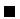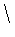Next: Meta-calls (Higher Order) Up: The Prolog Language Previous: Pruning Operators: Cut

# Meta-Logical Predicates

Prolog includes some meta-logical predicates (predicates which cannot be modeled in first-order logic) because they make programming simpler, and they allow the users to have more control on the program executions, controlling clause execution and restoring flexibility to programs using certain builtins. We are listing some of them in Table 4.5.

Table 4.5: Some meta-logical Prolog predicates
Name Meaning
var(X) X is currently a free variable
nonvar(X) X is not a free variable
ground(X) X is a term not containing variables

They never cause error, or instantiate variables, but the state of variables can be inspected safely. They do not have a first-order reading, since the ordering of the goals matters for them:

?- var(X), X = 3.

yes
?- X = 3, var(X).

noAlthough programs usually sort numbers, or strings, or similar entities, Prolog has a notion of a so-called standard order among all terms. This means that, apart from the arithmetical order among numbers, any two terms (being them atoms, structures, variables, numbers, etc.), can be compared for equality, disequality, and precedence. Of course this order is somewhat arbitrary, but it is usually adequate for most applications--in fact, since we are imposing an ordering among heterogeneous entities, either this ordering is highly application-dependent, or it is used just for the sake of keeping those items sorted somehow. Standard order checking primitives are shown in Table 4.6. It is interesting to note that the identity comparison == / 2 compares variables without binding them. In fact, it does not report two variables being equal unless they are the same:

?- X == Y.

no
?- X = Y, X == Y.
Y = X ?

yes


Example 4.2   The following chunk of code can maintain an ordered list of terms, possibly including variables, numbers, atoms, etc. insert(List, Term, NewList) adds Term to List (ordered and without repetitions) to obtain NewList, also ordered without repetitions.

insert([], It, [It]).
insert([H|T], It, [H|T]):- H == It.
insert([H|T], It, [It, H|T]):- H @> It.
insert([H|T], It, [H|NewT]) :-
H @< It,
insert(T, It, NewT).


Note the use of == / 2 to check for identity, so that variables can be added without further instantiating them.Example 4.3   In Sections 3.14 and 3.15 we assumed a precedes/2 implementation-dependent predicate. For a general sorted tree implementation, the standard order predicate @< / 2 can be used.Table 4.6: Predicates which implement standard order
Name Meaning
== / 2 Identity of terms== / 2 Nonidentity of terms
@> / 2, @>= / 2, @< / 2, @=< / 2 Precedence comparison

The order among terms is the following:

1.
Variables, oldest first. The order is not related to the names of variables.
2.
Floats, in numeric order.
3.
Integers, in numeric order.
4.
Atoms, in alphabetical order.
5.
Structures, ordered first by arity, then by the name of the principal functor, then by the arguments left-to-right.

Example 4.4   In Example 4.1 we saw how to check a term for unifiability of one of its subterms with another given term. We might not want to instantiate any term. This is achieved by changing the two clauses of subterm/2 to:

subterm(Sub,Term):-  Sub == Term.
subterm(Sub,Term):-
nonvar(Term),
functor(Term,F,N),
subterm(N,Sub,Term).Next: Meta-calls (Higher Order) Up: The Prolog Language Previous: Pruning Operators: Cut
MCL
1998-12-03